# RS Aggarwal Solutions for Class 12 Maths Chapter 1: Relations

In Class 11, the students have learnt about the Cartesian product of sets. Now in this chapter, students will get a brief idea about the relation in a set. Here, students who find it difficult in solving problems using RS Aggarwal textbook can refer the solutions to ace the exam. We at BYJU’S have a set of faculty with vast experience in the education industry, designing answers based on the requirements of students.

The solutions are made available in PDF format so that students can boost their exam preparation. Scoring good marks in board exam is the ambition of many students and with the help of these solutions they come out with flying colours. Students can use RS Aggarwal Solutions for Class 12 Maths Chapter 1 Relations PDF from the links, which are given here.

## RS Aggarwal Solutions for Class 12 Maths Chapter 1: Relations Download PDF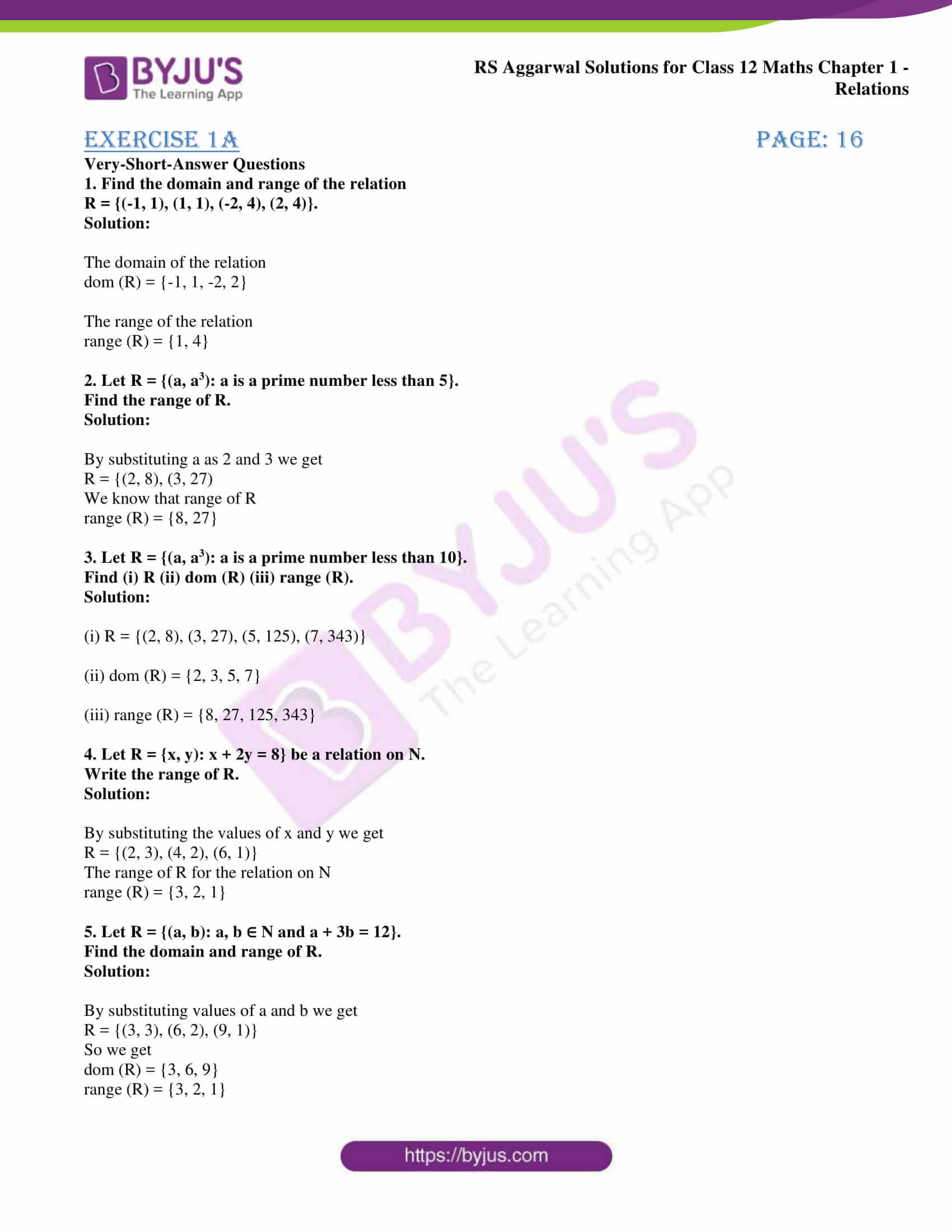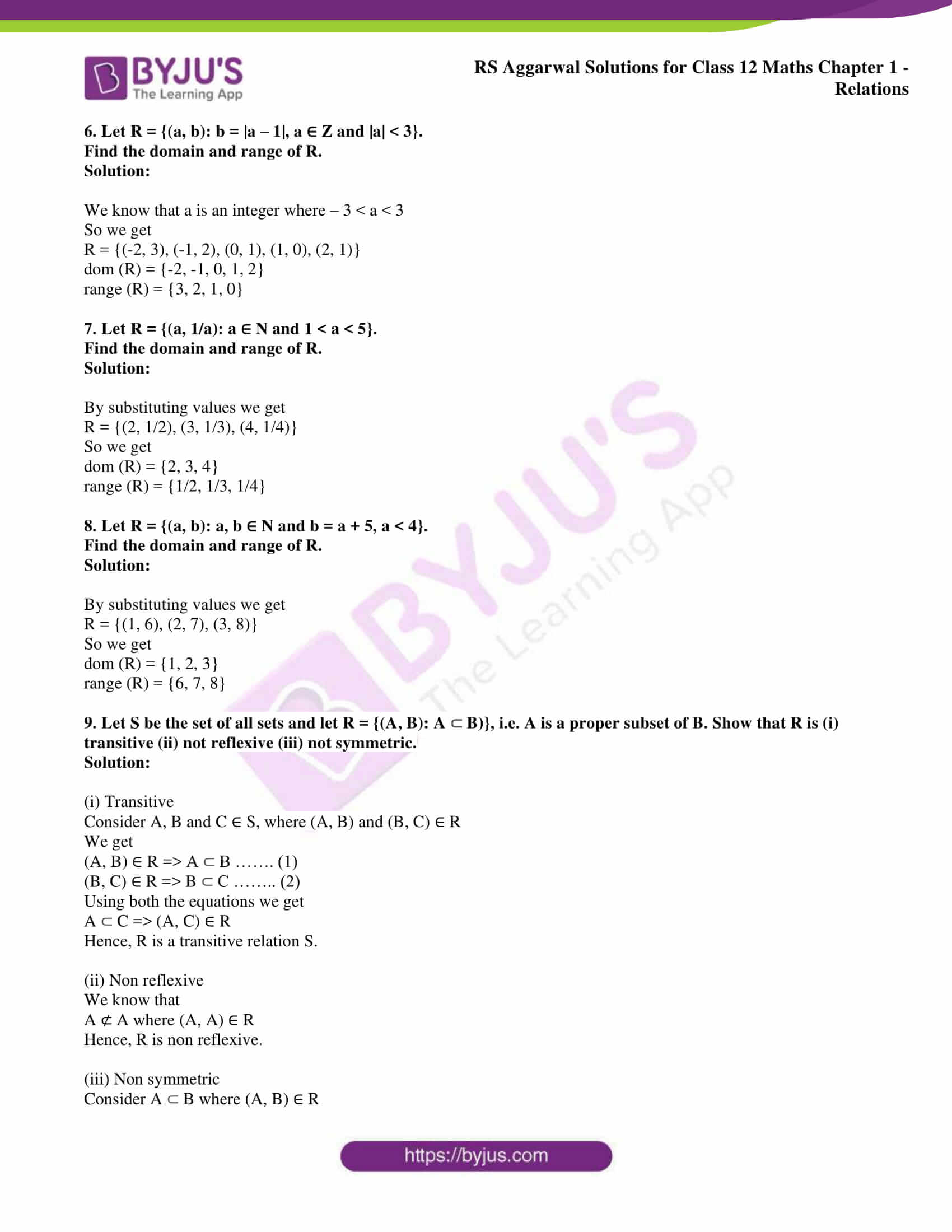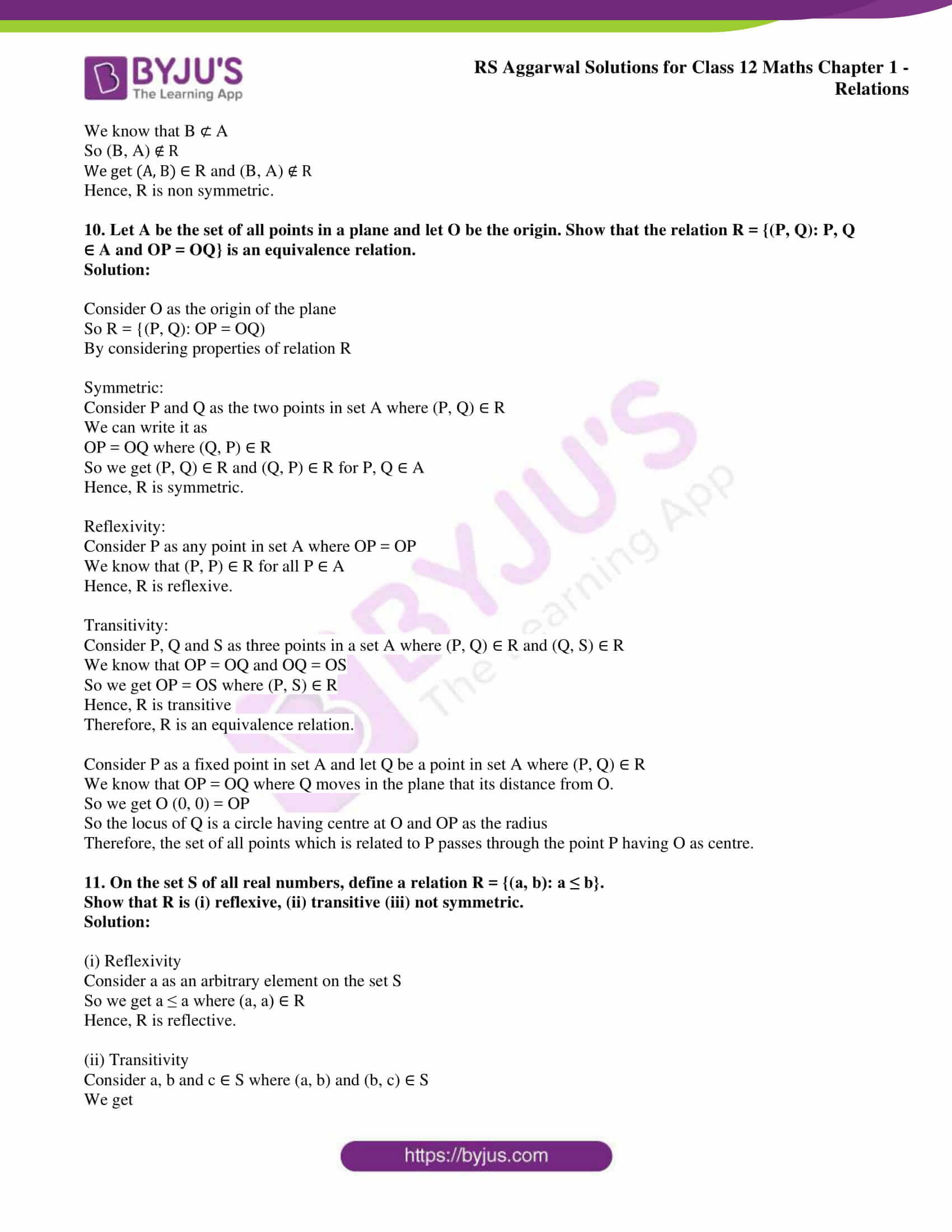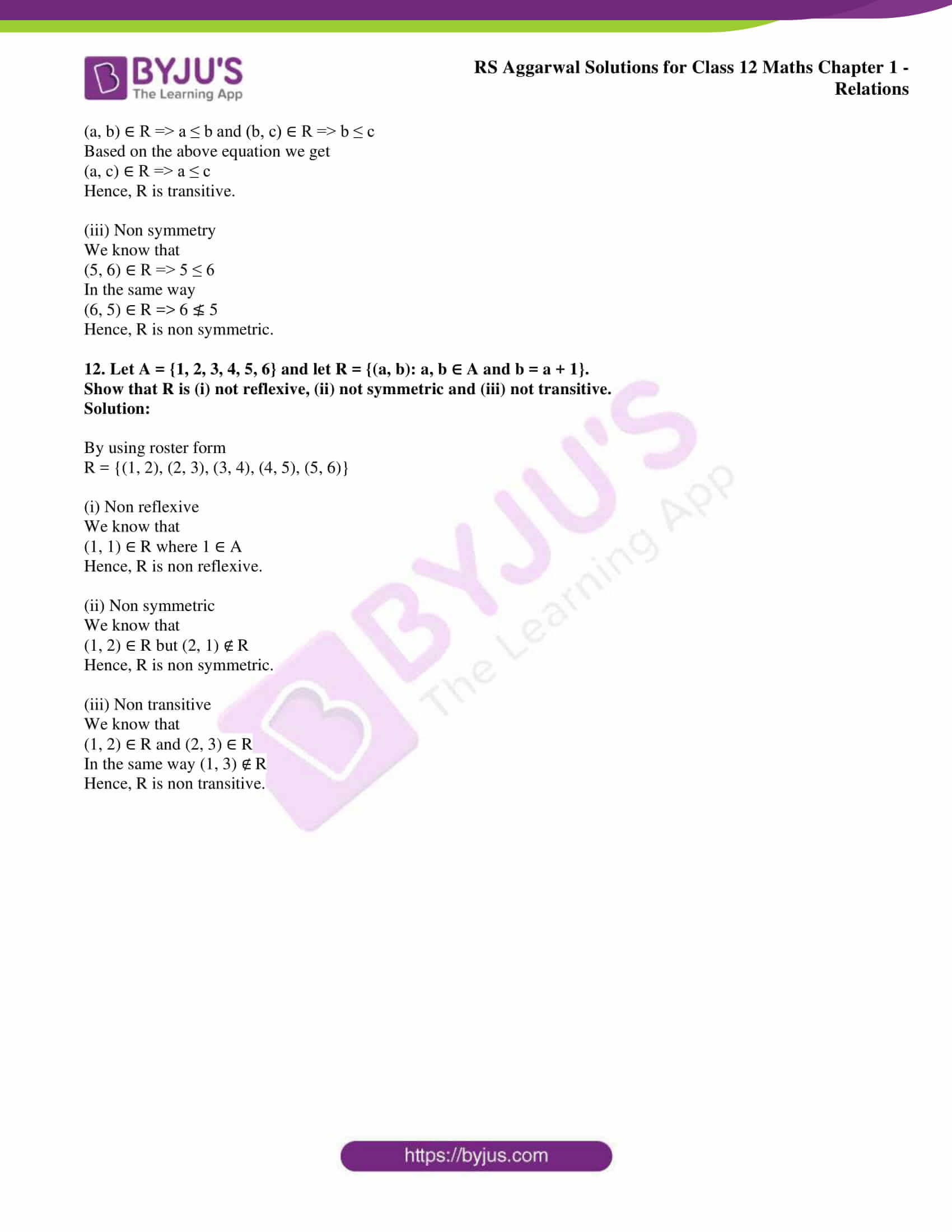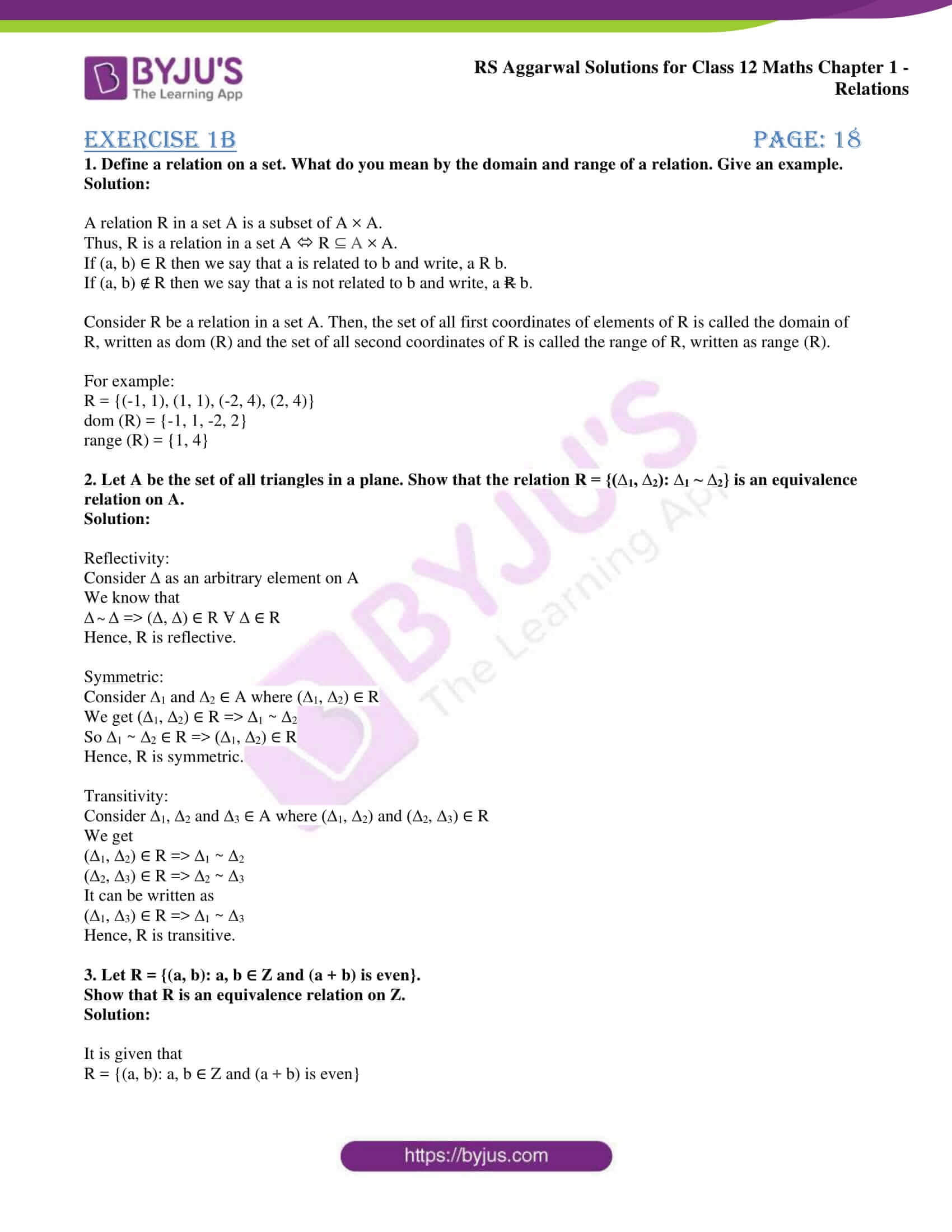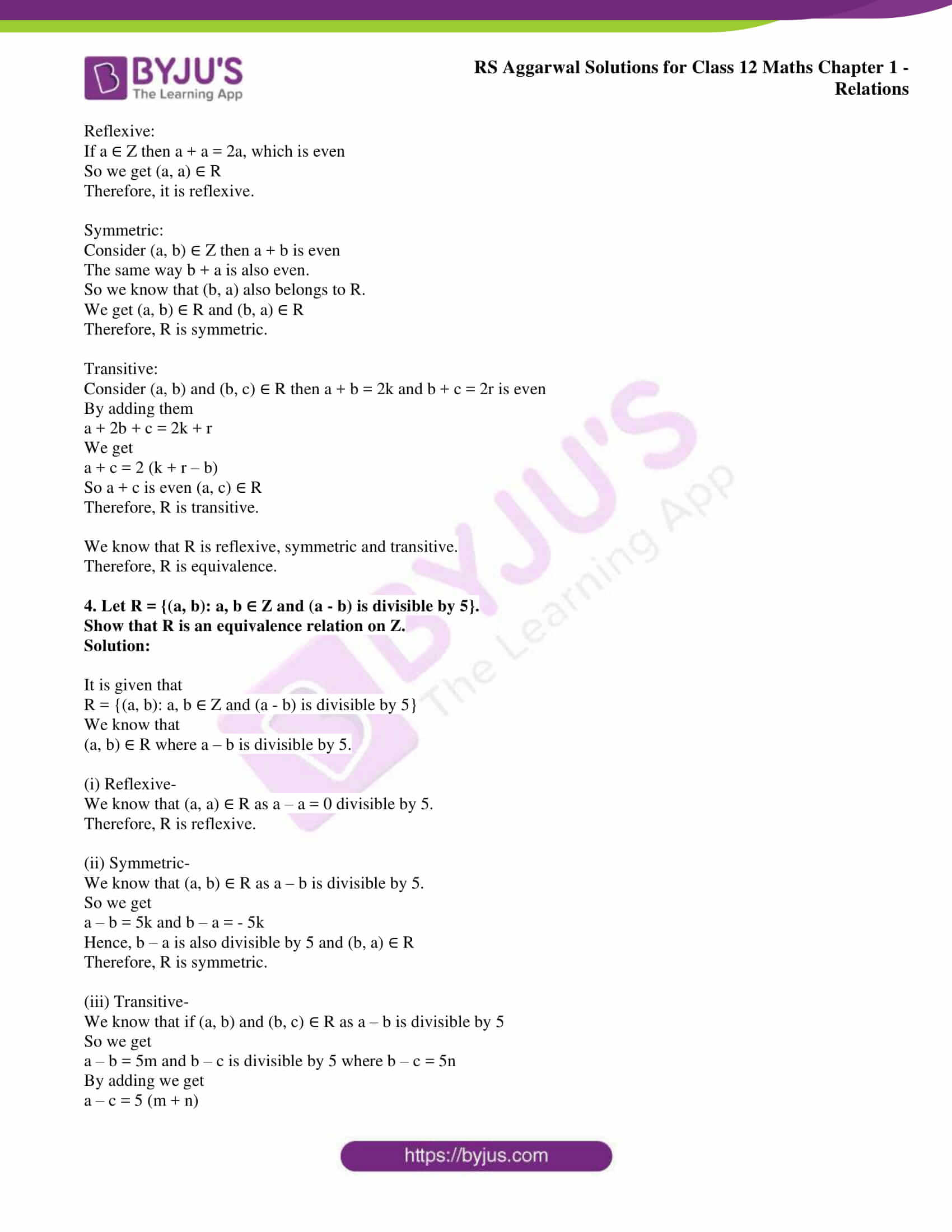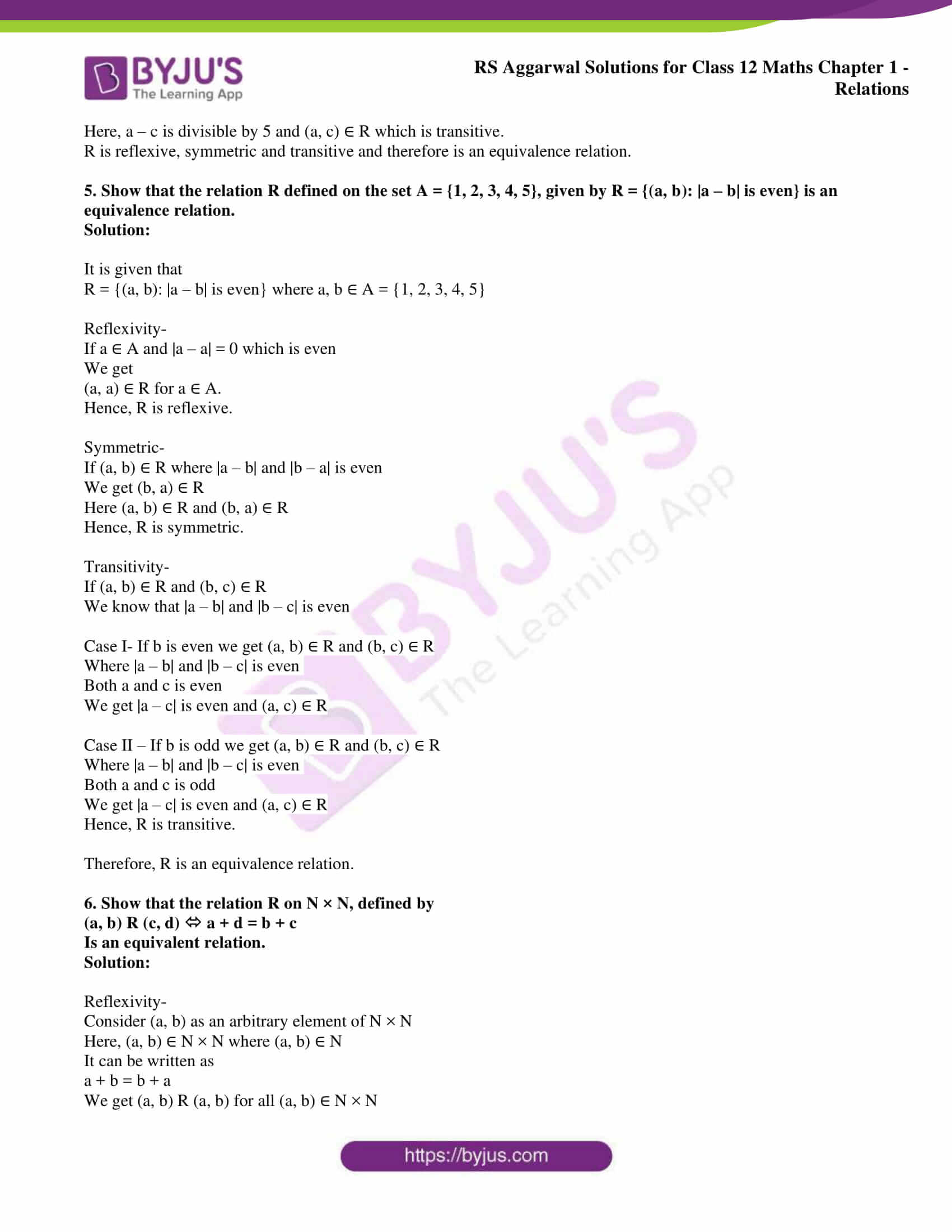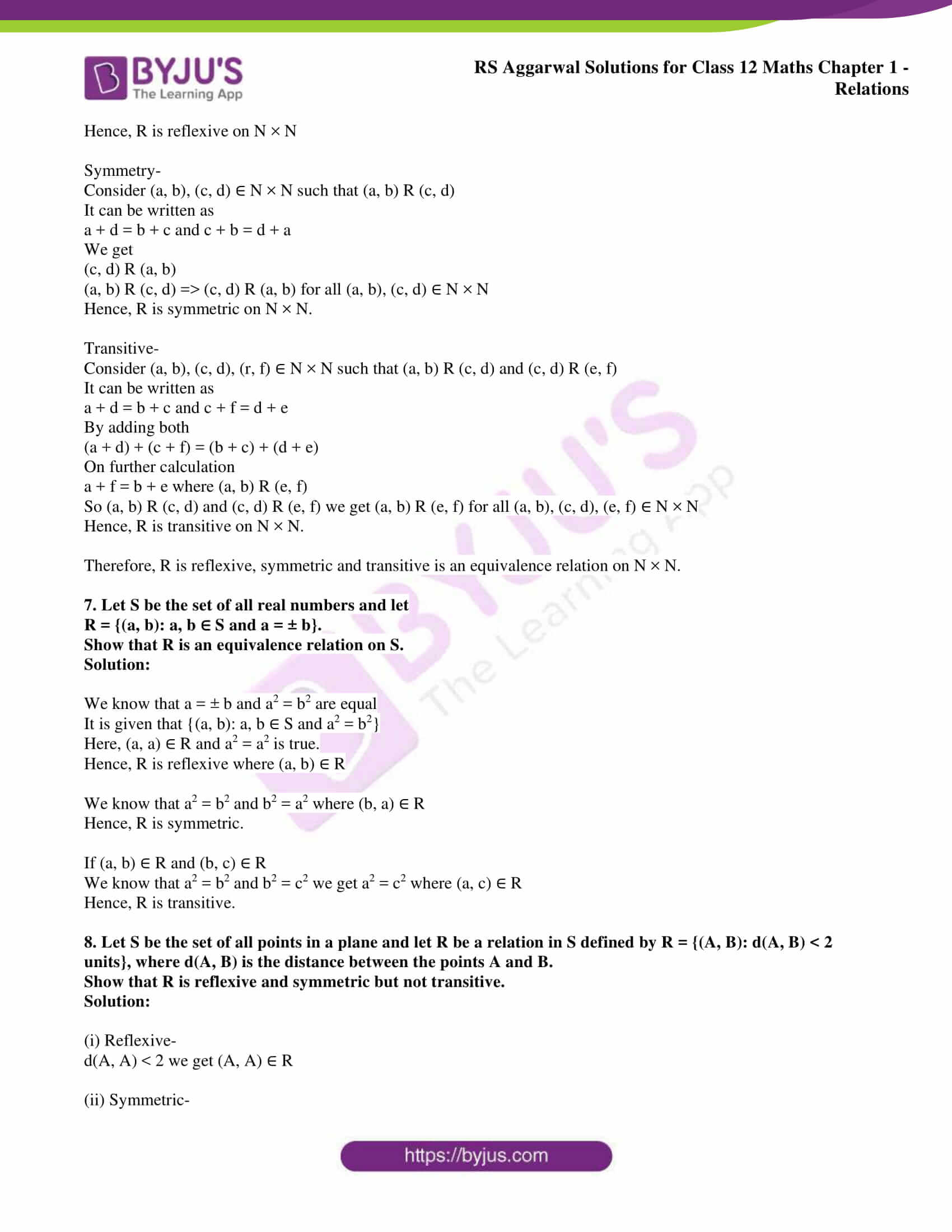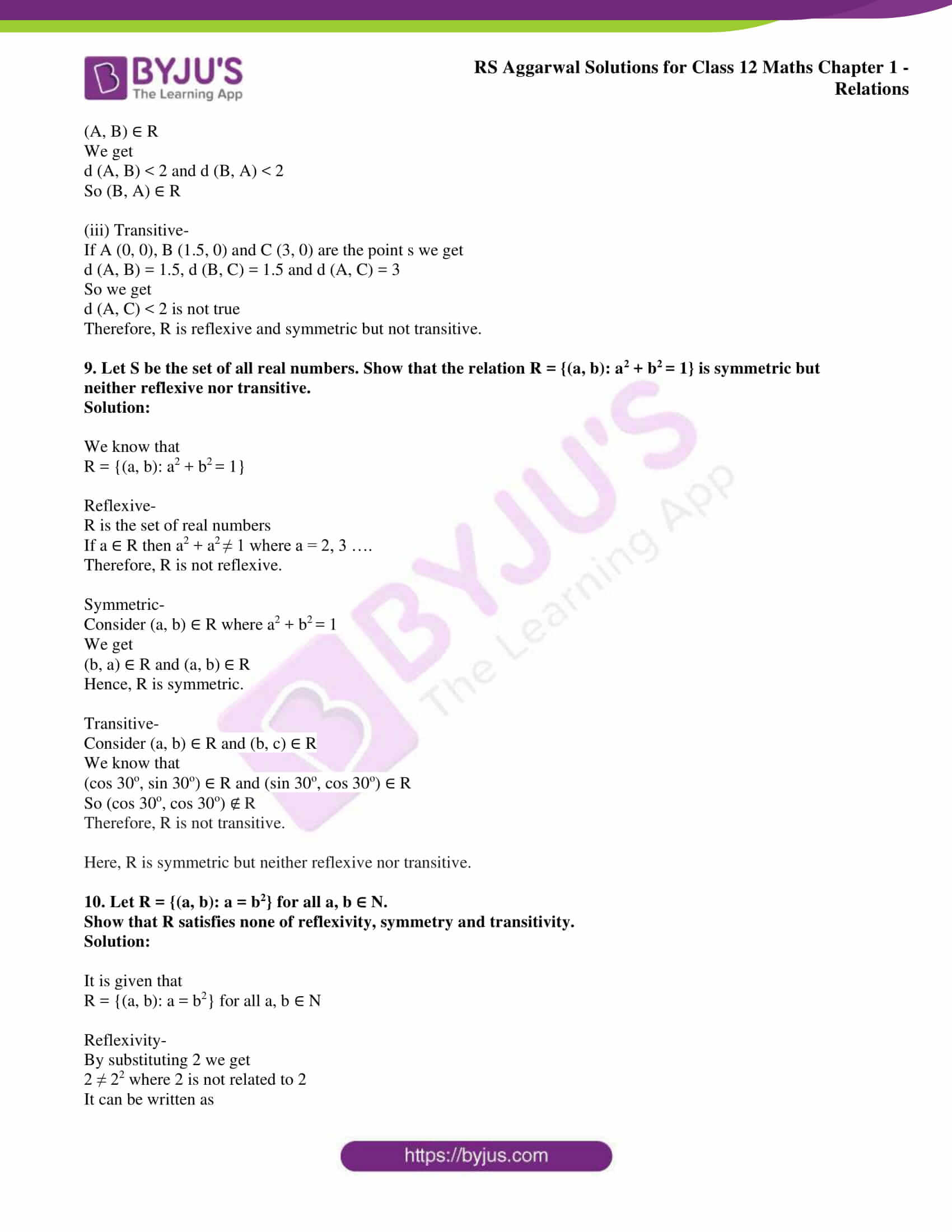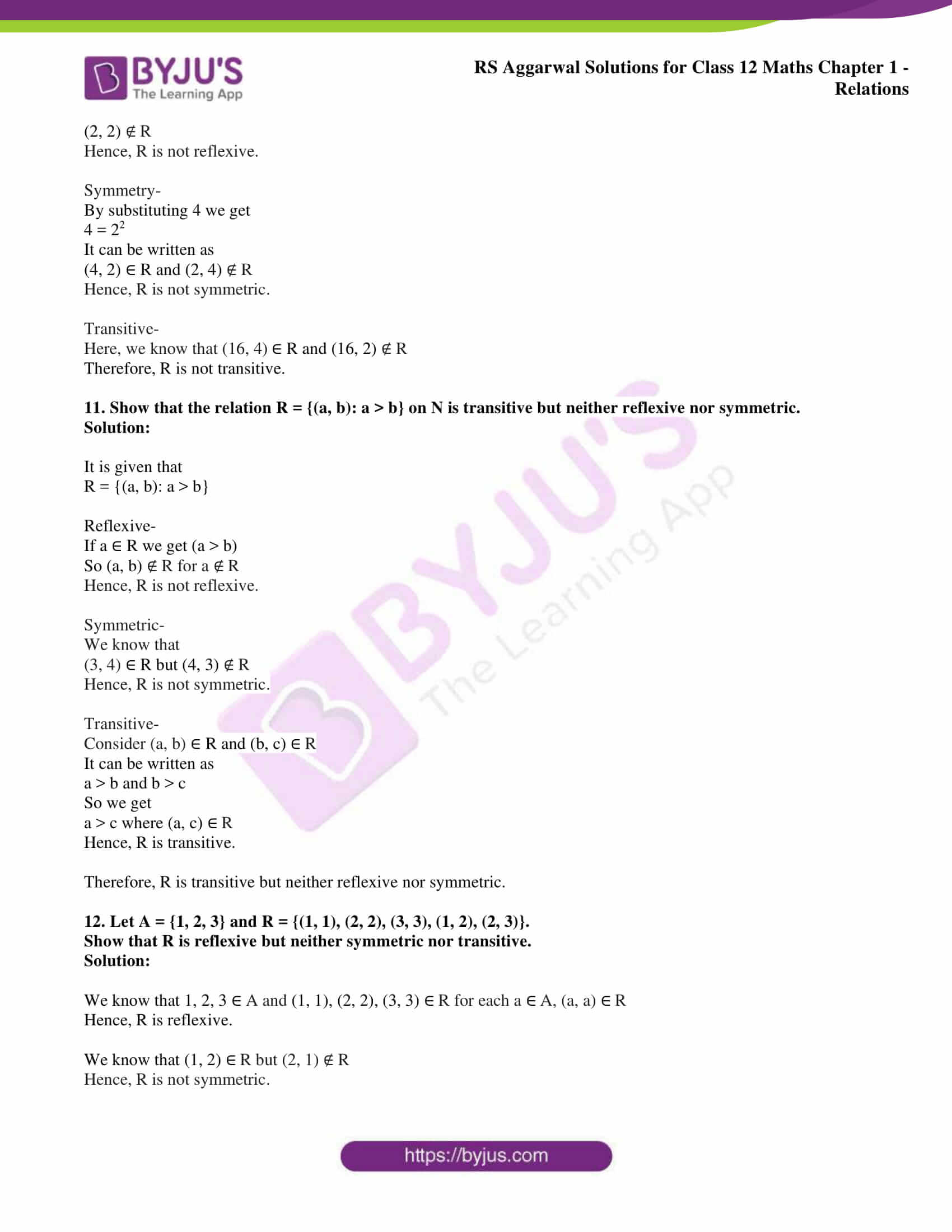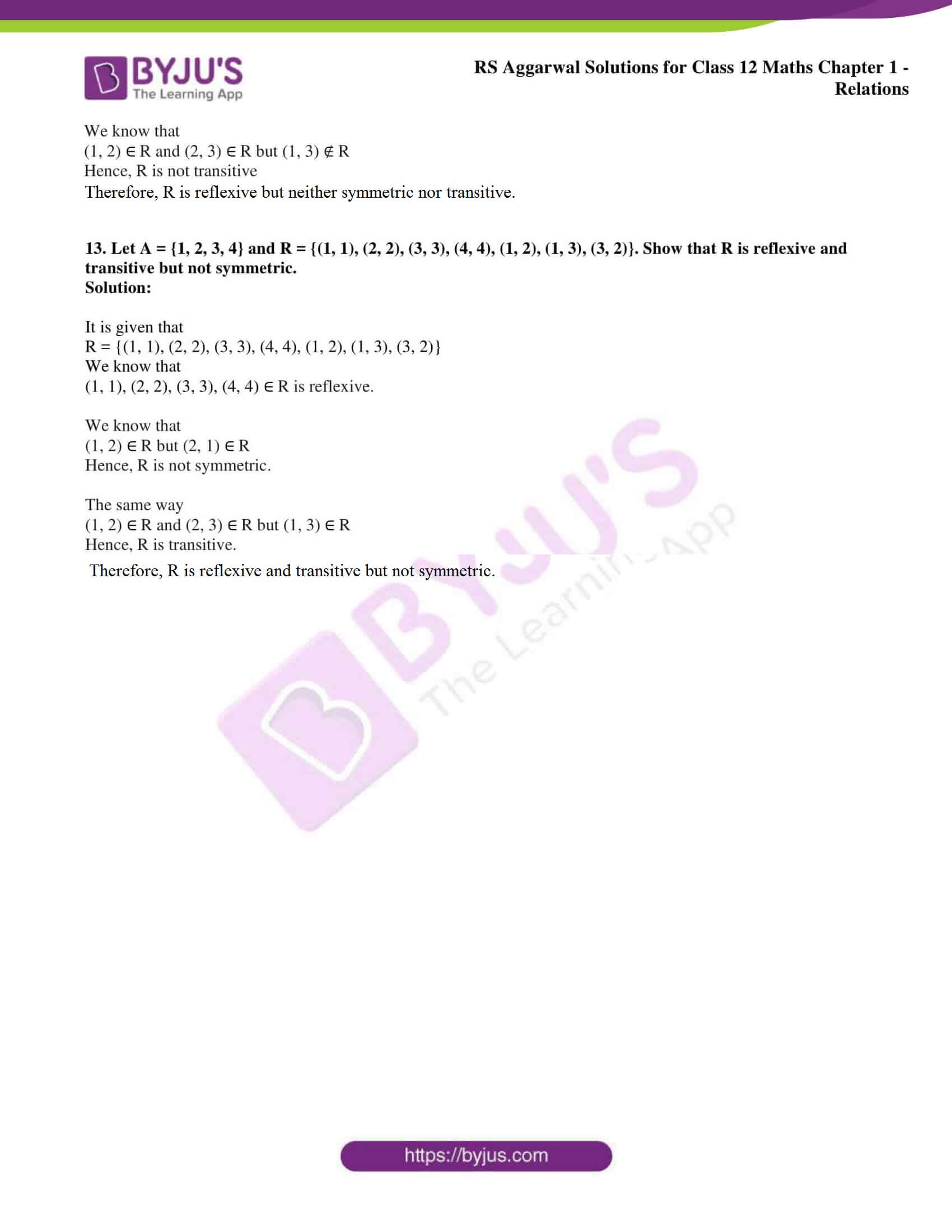### RS Aggarwal Solutions Class 12 Maths Chapter 1 – Relations

Exercise 1A Solutions

Exercise 1B Solutions

## Access RS Aggarwal Solutions for Class 12 Maths Chapter 1: Relations

Exercise 1A PAGE: 16

1. Find the domain and range of the relation

R = {(-1, 1), (1, 1), (-2, 4), (2, 4)}.

Solution:

The domain of the relation

dom (R) = {-1, 1, -2, 2}

The range of the relation

range (R) = {1, 4}

2. Let R = {(a, a3): a is a prime number less than 5}.

Find the range of R.

Solution:

By substituting a as 2 and 3 we get

R = {(2, 8), (3, 27)

We know that range of R

range (R) = {8, 27}

3. Let R = {(a, a3): a is a prime number less than 10}.

Find (i) R (ii) dom (R) (iii) range (R).

Solution:

(i) R = {(2, 8), (3, 27), (5, 125), (7, 343)}

(ii) dom (R) = {2, 3, 5, 7}

(iii) range (R) = {8, 27, 125, 343}

4. Let R = {x, y): x + 2y = 8} be a relation on N.

Write the range of R.

Solution:

By substituting the values of x and y we get

R = {(2, 3), (4, 2), (6, 1)}

The range of R for the relation on N

range (R) = {3, 2, 1}

5. Let R = {(a, b): a, b ∈ N and a + 3b = 12}.

Find the domain and range of R.

Solution:

By substituting values of a and b we get

R = {(3, 3), (6, 2), (9, 1)}

So we get

dom (R) = {3, 6, 9}

range (R) = {3, 2, 1}

6. Let R = {(a, b): b = |a – 1|, a ∈ Z and |a| < 3}.

Find the domain and range of R.

Solution:

We know that a is an integer where – 3 < a < 3

So we get

R = {(-2, 3), (-1, 2), (0, 1), (1, 0), (2, 1)}

dom (R) = {-2, -1, 0, 1, 2}

range (R) = {3, 2, 1, 0}

7. Let R = {(a, 1/a): a ∈ N and 1 < a < 5}.

Find the domain and range of R.

Solution:

By substituting values we get

R = {(2, 1/2), (3, 1/3), (4, 1/4)}

So we get

dom (R) = {2, 3, 4}

range (R) = {1/2, 1/3, 1/4}

8. Let R = {(a, b): a, b ∈ N and b = a + 5, a < 4}.

Find the domain and range of R.

Solution:

By substituting values we get

R = {(1, 6), (2, 7), (3, 8)}

So we get

dom (R) = {1, 2, 3}

range (R) = {6, 7, 8}

9. Let S be the set of all sets and let R = {(A, B): A ⊂ B)}, i.e. A is a proper subset of B. Show that R is (i) transitive (ii) not reflexive (iii) not symmetric.

Solution:

(i) Transitive

Consider A, B and C ∈ S, where (A, B) and (B, C) ∈ R

We get

(A, B) ∈ R => A ⊂ B ……. (1)

(B, C) ∈ R => B ⊂ C …….. (2)

Using both the equations we get

A ⊂ C => (A, C) ∈ R

Hence, R is a transitive relation S.

(ii) Non reflexive

We know that

A ⊄ A where (A, A) ∈ R

Hence, R is non reflexive.

(iii) Non symmetric

Consider A ⊂ B where (A, B) ∈ R

We know that B ⊄ A

So (B, A) ∉ R

We get (A, B) ∈ R and (B, A) ∉ R

Hence, R is non symmetric.

10. Let A be the set of all points in a plane and let O be the origin. Show that the relation R = {(P, Q): P, Q ∈ A and OP = OQ} is an equivalence relation.

Solution:

Consider O as the origin of the plane

So R = {(P, Q): OP = OQ)

By considering properties of relation R

Symmetric:

Consider P and Q as the two points in set A where (P, Q) ∈ R

We can write it as

OP = OQ where (Q, P) ∈ R

So we get (P, Q) ∈ R and (Q, P) ∈ R for P, Q ∈ A

Hence, R is symmetric.

Reflexivity:

Consider P as any point in set A where OP = OP

We know that (P, P) ∈ R for all P ∈ A

Hence, R is reflexive.

Transitivity:

Consider P, Q and S as three points in a set A where (P, Q) ∈ R and (Q, S) ∈ R

We know that OP = OQ and OQ = OS

So we get OP = OS where (P, S) ∈ R

Hence, R is transitive

Therefore, R is an equivalence relation.

Consider P as a fixed point in set A and let Q be a point in set A where (P, Q) ∈ R

We know that OP = OQ where Q moves in the plane that its distance from O.

So we get O (0, 0) = OP

So the locus of Q is a circle having centre at O and OP as the radius

Therefore, the set of all points which is related to P passes through the point P having O as centre.

11. On the set S of all real numbers, define a relation R = {(a, b): a ≤ b}.

Show that R is (i) reflexive, (ii) transitive (iii) not symmetric.

Solution:

(i) Reflexivity

Consider a as an arbitrary element on the set S

So we get a ≤ a where (a, a) ∈ R

Hence, R is reflective.

(ii) Transitivity

Consider a, b and c ∈ S where (a, b) and (b, c) ∈ S

We get

(a, b) ∈ R => a ≤ b and (b, c) ∈ R => b ≤ c

Based on the above equation we get

(a, c) ∈ R => a ≤ c

Hence, R is transitive.

(iii) Non symmetry

We know that

(5, 6) ∈ R => 5 ≤ 6

In the same way

(6, 5) ∈ R => 6 ≰ 5

Hence, R is non symmetric.

12. Let A = {1, 2, 3, 4, 5, 6} and let R = {(a, b): a, b ∈ A and b = a + 1}.

Show that R is (i) not reflexive, (ii) not symmetric and (iii) not transitive.

Solution:

By using roster form

R = {(1, 2), (2, 3), (3, 4), (4, 5), (5, 6)}

(i) Non reflexive

We know that

(1, 1) ∈ R where 1 ∈ A

Hence, R is non reflexive.

(ii) Non symmetric

We know that

(1, 2) ∈ R but (2, 1) ∉ R

Hence, R is non symmetric.

(iii) Non transitive

We know that

(1, 2) ∈ R and (2, 3) ∈ R

In the same way (1, 3) ∉ R

Hence, R is non transitive.

Exercise 1B page: 18

1. Define a relation on a set. What do you mean by the domain and range of a relation. Give an example.

Solution:

A relation R in a set A is a subset of A × A.

Thus, R is a relation in a set A R ⊆ A × A.

If (a, b) ∈ R then we say that a is related to b and write, a R b.

If (a, b) ∉ R then we say that a is not related to b and write, a b.

Consider R be a relation in a set A. Then, the set of all first coordinates of elements of R is called the domain of R, written as dom (R) and the set of all second coordinates of R is called the range of R, written as range (R).

For example:

R = {(-1, 1), (1, 1), (-2, 4), (2, 4)}

dom (R) = {-1, 1, -2, 2}

range (R) = {1, 4}

2. Let A be the set of all triangles in a plane. Show that the relation R = {(∆1, ∆2): ∆1 ~ ∆2} is an equivalence relation on A.

Solution:

Reflectivity:

Consider ∆ as an arbitrary element on A

We know that

∆ ~ ∆ => (∆, ∆) ∈ R Ɐ ∆ ∈ R

Hence, R is reflective.

Symmetric:

Consider ∆1 and ∆2 ∈ A where (∆1, ∆2) ∈ R

We get (∆1, ∆2) ∈ R => ∆1 ~ ∆2

So ∆1 ~ ∆2 ∈ R => (∆1, ∆2) ∈ R

Hence, R is symmetric.

Transitivity:

Consider ∆1, ∆2 and ∆3 ∈ A where (∆1, ∆2) and (∆2, ∆3) ∈ R

We get

(∆1, ∆2) ∈ R => ∆1 ~ ∆2

(∆2, ∆3) ∈ R => ∆2 ~ ∆3

It can be written as

(∆1, ∆3) ∈ R => ∆1 ~ ∆3

Hence, R is transitive.

3. Let R = {(a, b): a, b ∈ Z and (a + b) is even}.

Show that R is an equivalence relation on Z.

Solution:

It is given that

R = {(a, b): a, b ∈ Z and (a + b) is even}

Reflexive:

If a ∈ Z then a + a = 2a, which is even

So we get (a, a) ∈ R

Therefore, it is reflexive.

Symmetric:

Consider (a, b) ∈ Z then a + b is even

The same way b + a is also even.

So we know that (b, a) also belongs to R.

We get (a, b) ∈ R and (b, a) ∈ R

Therefore, R is symmetric.

Transitive:

Consider (a, b) and (b, c) ∈ R then a + b = 2k and b + c = 2r is even

a + 2b + c = 2k + r

We get

a + c = 2 (k + r – b)

So a + c is even (a, c) ∈ R

Therefore, R is transitive.

We know that R is reflexive, symmetric and transitive.

Therefore, R is equivalence.

4. Let R = {(a, b): a, b ∈ Z and (a – b) is divisible by 5}.

Show that R is an equivalence relation on Z.

Solution:

It is given that

R = {(a, b): a, b ∈ Z and (a – b) is divisible by 5}

We know that

(a, b) ∈ R where a – b is divisible by 5.

(i) Reflexive-

We know that (a, a) ∈ R as a – a = 0 divisible by 5.

Therefore, R is reflexive.

(ii) Symmetric-

We know that (a, b) ∈ R as a – b is divisible by 5.

So we get

a – b = 5k and b – a = – 5k

Hence, b – a is also divisible by 5 and (b, a) ∈ R

Therefore, R is symmetric.

(iii) Transitive-

We know that if (a, b) and (b, c) ∈ R as a – b is divisible by 5

So we get

a – b = 5m and b – c is divisible by 5 where b – c = 5n

a – c = 5 (m + n)

Here, a – c is divisible by 5 and (a, c) ∈ R which is transitive.

R is reflexive, symmetric and transitive and therefore is an equivalence relation.

5. Show that the relation R defined on the set A = {1, 2, 3, 4, 5}, given by R = {(a, b): |a – b| is even} is an equivalence relation.

Solution:

It is given that

R = {(a, b): |a – b| is even} where a, b ∈ A = {1, 2, 3, 4, 5}

Reflexivity-

If a ∈ A and |a – a| = 0 which is even

We get

(a, a) ∈ R for a ∈ A.

Hence, R is reflexive.

Symmetric-

If (a, b) ∈ R where |a – b| and |b – a| is even

We get (b, a) ∈ R

Here (a, b) ∈ R and (b, a) ∈ R

Hence, R is symmetric.

Transitivity-

If (a, b) ∈ R and (b, c) ∈ R

We know that |a – b| and |b – c| is even

Case I- If b is even we get (a, b) ∈ R and (b, c) ∈ R

Where |a – b| and |b – c| is even

Both a and c is even

We get |a – c| is even and (a, c) ∈ R

Case II – If b is odd we get (a, b) ∈ R and (b, c) ∈ R

Where |a – b| and |b – c| is even

Both a and c is odd

We get |a – c| is even and (a, c) ∈ R

Hence, R is transitive.

Therefore, R is an equivalence relation.

6. Show that the relation R on N × N, defined by

(a, b) R (c, d) a + d = b + c

Is an equivalent relation.

Solution:

Reflexivity-

Consider (a, b) as an arbitrary element of N × N

Here, (a, b) ∈ N × N where (a, b) ∈ N

It can be written as

a + b = b + a

We get (a, b) R (a, b) for all (a, b) ∈ N × N

Hence, R is reflexive on N × N

Symmetry-

Consider (a, b), (c, d) ∈ N × N such that (a, b) R (c, d)

It can be written as

a + d = b + c and c + b = d + a

We get

(c, d) R (a, b)

(a, b) R (c, d) => (c, d) R (a, b) for all (a, b), (c, d) ∈ N × N

Hence, R is symmetric on N × N.

Transitive-

Consider (a, b), (c, d), (r, f) ∈ N × N such that (a, b) R (c, d) and (c, d) R (e, f)

It can be written as

a + d = b + c and c + f = d + e

(a + d) + (c + f) = (b + c) + (d + e)

On further calculation

a + f = b + e where (a, b) R (e, f)

So (a, b) R (c, d) and (c, d) R (e, f) we get (a, b) R (e, f) for all (a, b), (c, d), (e, f) ∈ N × N

Hence, R is transitive on N × N.

Therefore, R is reflexive, symmetric and transitive is an equivalence relation on N × N.

7. Let S be the set of all real numbers and let

R = {(a, b): a, b ∈ S and a = ± b}.

Show that R is an equivalence relation on S.

Solution:

We know that a = ± b and a2 = b2 are equal

It is given that {(a, b): a, b ∈ S and a2 = b2}

Here, (a, a) ∈ R and a2 = a2 is true.

Hence, R is reflexive where (a, b) ∈ R

We know that a2 = b2 and b2 = a2 where (b, a) ∈ R

Hence, R is symmetric.

If (a, b) ∈ R and (b, c) ∈ R

We know that a2 = b2 and b2 = c2 we get a2 = c2 where (a, c) ∈ R

Hence, R is transitive.

8. Let S be the set of all points in a plane and let R be a relation in S defined by R = {(A, B): d(A, B) < 2 units}, where d(A, B) is the distance between the points A and B.

Show that R is reflexive and symmetric but not transitive.

Solution:

(i) Reflexive-

d(A, A) < 2 we get (A, A) ∈ R

(ii) Symmetric-

(A, B) ∈ R

We get

d (A, B) < 2 and d (B, A) < 2

So (B, A) ∈ R

(iii) Transitive-

If A (0, 0), B (1.5, 0) and C (3, 0) are the point s we get

d (A, B) = 1.5, d (B, C) = 1.5 and d (A, C) = 3

So we get

d (A, C) < 2 is not true

Therefore, R is reflexive and symmetric but not transitive.

9. Let S be the set of all real numbers. Show that the relation R = {(a, b): a2 + b2 = 1} is symmetric but neither reflexive nor transitive.

Solution:

We know that

R = {(a, b): a2 + b2 = 1}

Reflexive-

R is the set of real numbers

If a ∈ R then a2 + a2 ≠ 1 where a = 2, 3 ….

Therefore, R is not reflexive.

Symmetric-

Consider (a, b) ∈ R where a2 + b2 = 1

We get

(b, a) ∈ R and (a, b) ∈ R

Hence, R is symmetric.

Transitive-

Consider (a, b) ∈ R and (b, c) ∈ R

We know that

(cos 30o, sin 30o) ∈ R and (sin 30o, cos 30o) ∈ R

So (cos 30o, cos 30o) ∉ R

Therefore, R is not transitive.

Here, R is symmetric but neither reflexive nor transitive.

10. Let R = {(a, b): a = b2} for all a, b ∈ N.

Show that R satisfies none of reflexivity, symmetry and transitivity.

Solution:

It is given that

R = {(a, b): a = b2} for all a, b ∈ N

Reflexivity-

By substituting 2 we get

2 ≠ 22 where 2 is not related to 2

It can be written as

(2, 2) ∉ R

Hence, R is not reflexive.

Symmetry-

By substituting 4 we get

4 = 22

It can be written as

(4, 2) ∈ R and (2, 4) ∉ R

Hence, R is not symmetric.

Transitive-

Here, we know that (16, 4) ∈ R and (16, 2) ∉ R

Therefore, R is not transitive.

11. Show that the relation R = {(a, b): a > b} on N is transitive but neither reflexive nor symmetric.

Solution:

It is given that

R = {(a, b): a > b}

Reflexive-

If a ∈ R we get (a > b)

So (a, b) ∉ R for a ∉ R

Hence, R is not reflexive.

Symmetric-

We know that

(3, 4) ∈ R but (4, 3) ∉ R

Hence, R is not symmetric.

Transitive-

Consider (a, b) ∈ R and (b, c) ∈ R

It can be written as

a > b and b > c

So we get

a > c where (a, c) ∈ R

Hence, R is transitive.

Therefore, R is transitive but neither reflexive nor symmetric.

12. Let A = {1, 2, 3} and R = {(1, 1), (2, 2), (3, 3), (1, 2), (2, 3)}.

Show that R is reflexive but neither symmetric nor transitive.

Solution:

We know that 1, 2, 3 ∈ A and (1, 1), (2, 2), (3, 3) ∈ R for each a ∈ A, (a, a) ∈ R

Hence, R is reflexive.

We know that (1, 2) ∈ R but (2, 1) ∉ R

Hence, R is not symmetric.

We know that

(1, 2) ∈ R and (2, 3) ∈ R but (1, 3) ∉ R

Hence, R is not transitive

Therefore, R is reflexive but neither symmetric nor transitive.

13. Let A = {1, 2, 3, 4} and R = {(1, 1), (2, 2), (3, 3), (4, 4), (1, 2), (1, 3), (3, 2)}. Show that R is reflexive and transitive but not symmetric.

Solution:

It is given that

R = {(1, 1), (2, 2), (3, 3), (4, 4), (1, 2), (1, 3), (3, 2)}

We know that

(1, 1), (2, 2), (3, 3), (4, 4) ∈ R is reflexive.

We know that

(1, 2) ∈ R but (2, 1) ∈ R

Hence, R is not symmetric.

The same way

(1, 2) ∈ R and (2, 3) ∈ R but (1, 3) ∈ R

Hence, R is transitive.

Therefore, R is reflexive and transitive but not symmetric.